# Mathematics With Discounts & Taxes

in STEMGeeks4 months ago

Hi there. In this mathematics post I deal with mathematics with discounts and taxes. This topic is not as advanced as calculus but knowing how to deal with discounts and taxes is helpful for shopping, bargain hunting and for seeing if something is a good buy or not.

I do not really deal with finding the original price given the final price.

Math text images rendered in LaTeX and with QuickLaTeX.com.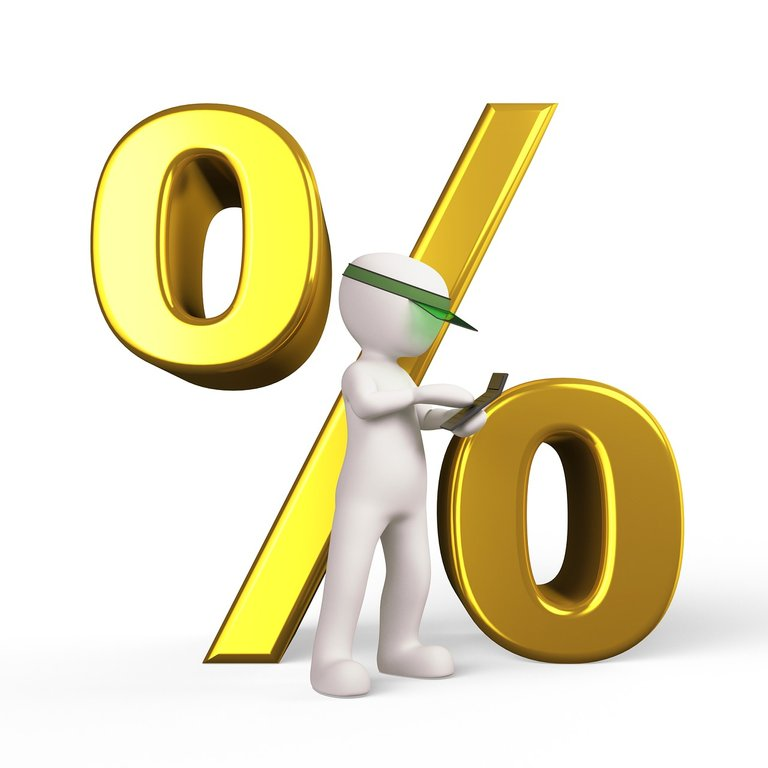Pixabay Image Source

## Sale Discounts For Paying Less Than The Original Price

Whenever there is a discount you pay less than the original price. In a more technical sense you pay less than 100% of the original price. If something is 10% off it would be 10% off the original price. This is also the same as paying 90% of the original price.

Example One

A winter jacket is priced at \$300. It is on sale for 10% off. What is the amount of the discount and what is the final price? Assume no tax.

The amount of the discount is 10% of \$300. Ten percent means ten out of one hundred which is equal to 0.10 in decimal form. This would be `0.10 x \$300 = \$30`. From the 10% off sale you would take \$30 off from the original price.

The final price would be `\$300 - \$30 = \$270`.

Example Two

A laptop is priced at \$1400. What is the final price of the laptop after a discount of 20%? Assume no tax.

For this question you do not need to obtain the amount of the discount. There is no need to do `0.20 x 1400`. To get the final price you can do this calculation: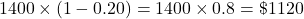Pixabay Image Source

Sales taxes add more to the original price in terms of cost. Different places have different tax rates when it comes to the purchases of items. There are a few places out there in the world where there are no taxes on purchased goods.

Example One

How much sales tax is paid on a \$2000 sofa with a 7% tax rate?

Compute 7% of \$2000. Note that seven percent is 0.07 in decimal form. The calculation would be `0.07 x 2000 = 140`. The tax paid is \$140.

Example Two

John's area has a sales tax of 8%. What would be the after-tax price on a \$200 pair of jeans?

The question just wants the after-tax price. There is no need to compute the tax amount. Add 8% as 0.08 to 1 and multiply that to two hundred dollars.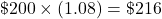Pixabay Image Source

## Combining Discounts & Taxes

When it comes to shopping and finding good deals you do encounter cases where there are discounts with taxes added on. Discounts are applied first and then the taxes are added on. (I could be wrong though.)

Example One

A large TV is originally prices at \$1200 with a 20% discount. Assume a tax rate at 12%. What is the final price for this TV?

Apply the 20% discount first. This is the same as paying 80% of \$1200. The keyword of means multiply in the math framework.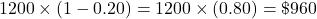From the discounted price of \$960 apply the 12 percent tax. This is multiplying by 1.12.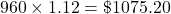You can combine the two steps above into one step. This is for going from the original price to the final price. For this you would multiply by 0.80 and by 1.12.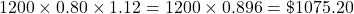Example Two

At a liquidation sale, Kayley finds a shirt priced at \$40 with a 50% discount. The tax rate is 8%. What is the final price of this shirt?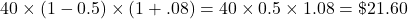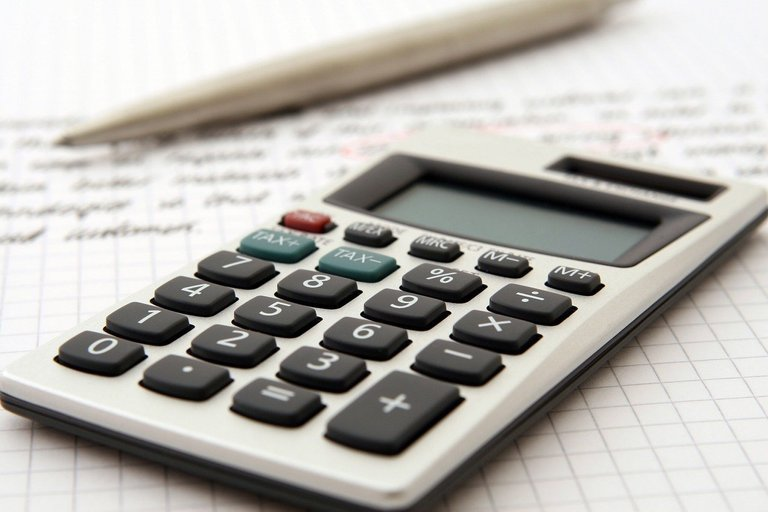Pixabay Image Source

## Multiple Discounts Case

Example One - No Tax

The price of a jacket is originally priced at \$219 with a 20% discount. In addition the store has another 10% discount on top of the 20% discount. The region also has no tax. What is the final price of this jacket?

With this type of case do not add the percentages together. The second 10% discount is applied to the price after the 20% discount. The first discount of 20% off means you pay 80% of the original price. The 10% discount means pay 90% of the discounted price.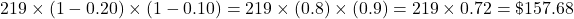The final price after these two discounts is \$157.68.

Example Two - Two Discounts & 10% Tax

A winter jacket has the original price of \$500. There is a discount of 10%. Jack has a membership card which gives him an additional 10% off his purchase. The tax rate in Jack's region is 10%. What is the final price of the jacket?

In this case do not cancel the 10% discount and 10% tax rate. This is important. You get the price after the first 10% discount then take another 10% off. The discounted price multiplied by 1.1 gives the final price of the jacket.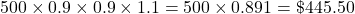The final price of the jacket is \$445.50.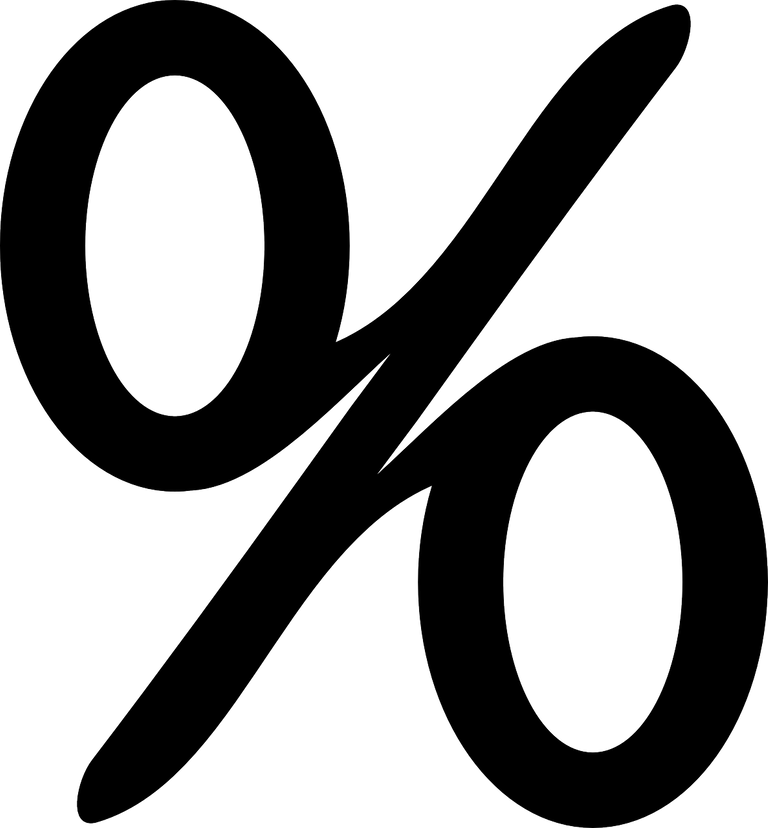Pixabay Image Source